The FREQ Procedure
 TABLES Statement

TABLES requests </ options> ;

The TABLES statement requests one-way to n-way frequency and crosstabulation tables and statistics for those tables.

If you omit the TABLES statement, PROC FREQ generates one-way frequency tables for all data set variables that are not listed in the other statements.

The following argument is required in the TABLES statement.

requests

specify the frequency and crosstabulation tables to produce. A request is composed of one variable name or several variable names separated by asterisks. To request a one-way frequency table, use a single variable. To request a two-way crosstabulation table, use an asterisk between two variables. To request a multiway table (an n-way table, where n>2), separate the desired variables with asterisks. The unique values of these variables form the rows, columns, and strata of the table. You can include up to 50 variables in a single multiway table request.

For two-way to multiway tables, the values of the last variable form the crosstabulation table columns, while the values of the next-to-last variable form the rows. Each level (or combination of levels) of the other variables forms one stratum. PROC FREQ produces a separate crosstabulation table for each stratum. For example, a specification of A*B*C*D in a TABLES statement produces k tables, where k is the number of different combinations of values for A and B. Each table lists the values for C down the side and the values for D across the top.

You can use multiple TABLES statements in the PROC FREQ step. PROC FREQ builds all the table requests in one pass of the data, so that there is essentially no loss of efficiency. You can also specify any number of table requests in a single TABLES statement. To specify multiple table requests quickly, use a grouping syntax by placing parentheses around several variables and joining other variables or variable combinations. For example, the statements shown in Table 3.8 illustrate grouping syntax.

Table 3.8 Grouping Syntax

Request

Equivalent to

tables A*(B C);

tables A*B    A*C;

tables (A B)*(C D);

tables A*C    B*C    A*D    B*D;

tables (A B C)*D;

tables A*D    B*D    C*D;

tables A – – C;

tables A    B    C;

tables (A – – C)*D;

tables A*D    B*D    C*D;

The TABLES statement variables are one or more variables from the DATA= input data set. These variables can be either character or numeric, but the procedure treats them as categorical variables. PROC FREQ uses the formatted values of the TABLES variable to determine the categorical variable levels. So if you assign a format to a variable with a FORMAT statement, PROC FREQ formats the values before dividing observations into the levels of a frequency or crosstabulation table. See the discussion of the FORMAT procedure in the Base SAS Procedures Guide and the discussions of the FORMAT statement and SAS formats in SAS Language Reference: Dictionary.

If you use PROC FORMAT to create a user-written format that combines missing and nonmissing values into one category, PROC FREQ treats the entire category of formatted values as missing. See the discussion in the section Grouping with Formats for more information.

The frequency or crosstabulation table lists the values of both character and numeric variables in ascending order based on internal (unformatted) variable values unless you change the order with the ORDER= option. To list the values in ascending order by formatted value, use ORDER=FORMATTED in the PROC FREQ statement.

### Without Options

If you request a one-way frequency table for a variable without specifying options, PROC FREQ produces frequencies, cumulative frequencies, percentages of the total frequency, and cumulative percentages for each value of the variable. If you request a two-way or an n-way crosstabulation table without specifying options, PROC FREQ produces crosstabulation tables that include cell frequencies, cell percentages of the total frequency, cell percentages of row frequencies, and cell percentages of column frequencies. The procedure excludes observations with missing values from the table but displays the total frequency of missing observations below each table.

### Options

Table 3.9 lists the options available in the TABLES statement. Descriptions follow in alphabetical order.

Table 3.9 TABLES Statement Options

Option

Description

Control Statistical Analysis

Requests tests and measures of classification agreement

Requests tests and measures of association produced by CHISQ,

MEASURES, and CMH

Sets the confidence level for confidence limits

Requests Tarone’s adjustment for the Breslow-Day test

Requests binomial proportion, confidence limits, and tests

for one-way tables

Requests BINOMIAL statistics with a continuity correction

Requests chi-square tests and measures based on chi-square

Requests confidence limits for the MEASURES statistics

Requests all Cochran-Mantel-Haenszel statistics

Requests CMH correlation statistic, adjusted odds ratios,

and adjusted relative risks

Requests CMH correlation and row mean scores (ANOVA)

statistics, adjusted odds ratios, and adjusted relative risks

Specifies convergence criterion for polychoric correlation

Requests Fisher’s exact test for tables larger than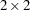Requests Gail-Simon test for qualitative interactions

Requests Jonckheere-Terpstra test

Specifies maximum number of iterations for polychoric correlation

Requests measures of association

Treats missing values as nonmissing

Requests polychoric correlation

Requests relative risk measures fortables

Requests risks and risk differences fortables

Requests RISKDIFF statistics with a continuity correction

Specifies the type of row and column scores

Specifies expected frequencies for one-way chi-square test

Specifies expected proportions for one-way chi-square test

Requests Cochran-Armitage test for trend

Control Additional Table Information

Displays cell contributions to the Pearson chi-square statistic

Displays cumulative column percentages

Displays deviations of cell frequencies from expected values

Displays expected cell frequencies

Displays missing value frequencies

Includes all possible combinations of variable levels

in LIST and OUT=

Displays percentages of total frequency for n-way tables (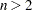)

Specifies the contents label for crosstabulation tables

Displays crosstabulation tables in ODS column format

Formats the frequencies in crosstabulation tables

Displays two-way to n-way tables in list format

Suppresses display of column percentages

Suppresses display of cumulative frequencies and percentages

Suppresses display of frequencies

Suppresses display of percentages

Suppresses display of crosstabulation tables but displays statistics

Suppresses display of row percentages

Suppresses zero frequency levels in CROSSLIST, LIST and OUT=

Suppresses log warning message for the chi-square test

Displays kappa coefficient weights

Displays row and column scores

Produce Statistical Graphics

Requests plots from ODS Graphics

Create an Output Data Set

Names an output data set to contain frequency counts

Includes cumulative frequencies and percentages

in the output data set for one-way tables

Includes expected frequencies in the output data set

Includes row, column, and two-way table percentages

in the output data set

You can specify the following options in a TABLES statement.

AGREE <(WT=FC)>

requests tests and measures of classification agreement for square tables. The AGREE option provides McNemar’s test fortables and Bowker’s test of symmetry for square tables with more than two response categories. The AGREE option also produces the simple kappa coefficient, the weighted kappa coefficient, their asymptotic standard errors, and their confidence limits. When there are multiple strata, the AGREE option provides overall simple and weighted kappas as well as tests for equal kappas among strata. When there are multiple strata and two response categories, PROC FREQ computes Cochran’s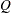test. See the section Tests and Measures of Agreement for details about these statistics.

If you specify the WT=FC option in parentheses following the AGREE option, PROC FREQ uses Fleiss-Cohen weights to compute the weighted kappa coefficient. By default, PROC FREQ uses Cicchetti-Allison weights. See the section Weighted Kappa Coefficient for details. You can specify the PRINTKWT option to display the kappa coefficient weights.

AGREE statistics are computed only for square tables, where the number of rows equals the number of columns. If your table is not square due to observations with zero weights, you can specify the ZEROS option in the WEIGHT statement to include these observations. For more details, see the section Tables with Zero Rows and Columns.

You can use the TEST statement to request asymptotic tests for the simple and weighted kappa coefficients. You can request exact p-values for the simple and weighted kappa coefficient tests, as well as for McNemar’s test, by specifying the corresponding options in the EXACT statement. See the section Exact Statistics for more information.

ALL

requests all of the tests and measures that are computed by the CHISQ, MEASURES, and CMH options. The number of CMH statistics computed can be controlled by the CMH1 and CMH2 options.

ALPHA=specifies the level of confidence limits. The value ofmust be between 0 and 1, and the default is 0.05. A confidence level ofproduces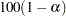% confidence limits. The default of ALPHA=0.05 produces 95% confidence limits.

ALPHA= applies to confidence limits requested by TABLES statement options. There is a separate ALPHA= option in the EXACT statement that sets the level of confidence limits for Monte Carlo estimates of exact p-values, which are requested in the EXACT statement.

BDT

requests Tarone’s adjustment in the Breslow-Day test for homogeneity of odds ratios. (You must specify the CMH option to compute the Breslow-Day test.) See the section Breslow-Day Test for Homogeneity of the Odds Ratios for more information.

BINOMIAL <(binomial-options)>

requests the binomial proportion for one-way tables. When you specify the BINOMIAL option, PROC FREQ also provides the asymptotic standard error, asymptotic (Wald) and exact (Clopper-Pearson) confidence limits, and the asymptotic equality test for the binomial proportion by default.

You can specify binomial-options inside the parentheses following the BINOMIAL option. The LEVEL= binomial-option identifies the variable level for which to compute the proportion. If you do not specify LEVEL=, PROC FREQ computes the proportion for the first level that appears in the output. The P= binomial-option specifies the null proportion for the binomial tests. If you do not specify P=, PROC FREQ uses P=0.5 by default.

You can also specify binomial-options to request confidence limits and tests of noninferiority, superiority, and equivalence for the binomial proportion. Table 3.10 summarizes the binomial-options.

Available confidence limits for the binomial proportion include Agresti-Coull, exact (Clopper-Pearson), Jeffreys, Wald, and Wilson (score) confidence limits. You can specify more than one type of binomial confidence limits in the same analysis. If you do not specify any confidence limit requests with binomial-options, PROC FREQ computes Wald asymptotic confidence limits and exact (Clopper-Pearson) confidence limits by default. The ALPHA= option determines the confidence level, and the default of ALPHA=0.05 produces 95% confidence limits for the binomial proportion.

To request exact tests for the binomial proportion, specify the BINOMIAL option in the EXACT statement. PROC FREQ then computes exact p-values for all binomial tests that you request with binomial-options, which can include tests of noninferiority, superiority, and equivalence, as well as the test of equality.

See the section Binomial Proportion for details.

Table 3.10 BINOMIAL Options

Task

Binomial-Option

Specify the variable level

Specify the null proportion

Request a continuity correction

Request confidence limits

Request tests

Specify the test margin

Specify the test variance

You can specify the following binomial-options inside parentheses following the BINOMIAL option.

AGRESTICOULL | AC

requests Agresti-Coull confidence limits for the binomial proportion. See the section Agresti-Coull Confidence Limits for details.

ALL

requests all available types of confidence limits for the binomial proportion. These include the following: Agresti-Coull, exact (Clopper-Pearson), Jeffreys, Wald, and Wilson (score) confidence limits.

CORRECT

includes a continuity correction in the asymptotic Wald confidence limits and tests. The CORRECT binomial-option has the same effect as the BINOMIALC option.

EQUIV | EQUIVALENCE

requests a test of equivalence for the binomial proportion. See the section Equivalence Test for details. You can specify the equivalence test margins, the null proportion, and the variance type with the MARGIN=, P=, and VAR= binomial-options, respectively.

EXACT
CLOPPERPEARSON

requests exact (Clopper-Pearson) confidence limits for the binomial proportion. See the section Exact (Clopper-Pearson) Confidence Limits for details. If you do not request any binomial confidence limits by specifying binomial-options, PROC FREQ produces Wald and exact (Clopper-Pearson) confidence limits by default.

JEFFREYS | J

requests Jeffreys confidence limits for the binomial proportion. See the section Jeffreys Confidence Limits for details.

LEVEL=level-number | 'level-value'

specifies the variable level for the binomial proportion. By default, PROC FREQ computes the proportion of observations for the first variable level that appears in the output. To request a different level, use LEVEL=level-number or LEVEL='level-value', where level-number is the variable level’s number or order in the output, and level-value is the formatted value of the variable level. The value of level-number must be a positive integer. You must enclose level-value in single quotes.

MARGIN=value | (lower,upper)

specifies the margin for the noninferiority, superiority, and equivalence tests, which you request with the NONINF, SUP, and EQUIV binomial-options, respectively. If you do not specify MARGIN=, PROC FREQ uses a margin of 0.2 by default.

For noninferiority and superiority tests, specify a single value for the MARGIN= option. The MARGIN= value must be a positive number. You can specify value as a number between 0 and 1. Or you can specify value in percentage form as a number between 1 and 100, and PROC FREQ converts that number to a proportion. The procedure treats the value 1 as 1%.

For noninferiority and superiority tests, the test limits must be between 0 and 1. The limits are determined by the null proportion value (which you can specify with the P= binomial-option) and by the margin value. The noninferiority limit equals the null proportion minus the margin. By default, the null proportion equals 0.5 and the margin equals 0.2, which gives a noninferiority limit of 0.3. The superiority limit equals the null proportion plus the margin, which is 0.7 by default.

For an equivalence test, you can specify a single MARGIN= value, or you can specify both lower and upper values. If you specify a single MARGIN= value, it must be a positive number, as described previously. If you specify a single MARGIN= value for an equivalence test, PROC FREQ uses –value as the lower margin and value as the upper margin for the test. If you specify both lower and upper values for an equivalence test, you can specify them in proportion form as numbers between –1 or 1. Or you can specify them in percentage form as numbers between –100 and 100, and PROC FREQ converts the numbers to proportions. The value of lower must be less than the value of upper.

The equivalence limits must be between 0 and 1. The equivalence limits are determined by the null proportion value (which you can specify with the P= binomial-option) and by the margin values. The lower equivalence limit equals the null proportion plus the lower margin. By default, the null proportion equals 0.5 and the lower margin equals –0.2, which gives a lower equivalence limit of 0.3. The upper equivalence limit equals the null proportion plus the upper margin, which is 0.7 by default.

See the sections Noninferiority Test and Equivalence Test for details.

NONINF | NONINFERIORITY

requests a test of noninferiority for the binomial proportion. See the section Noninferiority Test for details. You can specify the noninferiority test margin, the null proportion, and the variance type with the MARGIN=, P=, and VAR= binomial-options, respectively.

P=value

specifies the null hypothesis proportion for the binomial tests. If you omit the P= option, PROC FREQ uses 0.5 as the null proportion. The null proportion value must be a positive number. You can specify value as a number between 0 and 1. Or you can specify value in percentage form as a number between 1 and 100, and PROC FREQ converts that number to a proportion. The procedure treats the value 1 as 1%.

SUP | SUPERIORITY

requests a test of superiority for the binomial proportion. See the section Superiority Test for details. You can specify the superiority test margin, the null proportion, and the variance type with the MARGIN=, P=, and VAR= binomial-options, respectively.

VAR=SAMPLE | NULL

specifies the type of variance estimate to use for the tests of noninferiority, superiority, and equivalence. The default is VAR=SAMPLE, which estimates the variance from the sample proportion. VAR=NULL uses the null proportion to compute the variance. See the sections Noninferiority Test and Equivalence Test for details.

WALD

requests Wald confidence limits for the binomial proportion. See the section Wald Confidence Limits for details. If you do not request any binomial confidence limits by specifying binomial-options, PROC FREQ produces Wald and exact (Clopper-Pearson) confidence limits by default.

WILSON | W
SCORE

requests Wilson confidence limits for the binomial proportion. These are also known as score confidence limits. See the section Wilson (Score) Confidence Limits for details.

BINOMIALC <(binomial-options)>

requests the BINOMIAL option statistics for one-way tables, and includes a continuity correction in the asymptotic Wald confidence limits and tests. The BINOMIAL option statistics include the binomial proportion, its asymptotic standard error, asymptotic (Wald) and exact (Clopper-Pearson) confidence limits, and the asymptotic equality test for the binomial proportion by default. The binomial-options available with the BINOMIALC option are the same as those available with BINOMIAL. See the description of the BINOMIAL option for details.

CELLCHI2

displays each crosstabulation table cell’s contribution to the total Pearson chi-square statistic. The cell contribution is computed as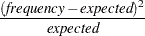where frequency is the table cell frequency or count and expected is the expected cell frequency, which is computed under the null hypothesis that the row and column variables are independent. See the section Pearson Chi-Square Test for Two-Way Tables for details.

The CELLCHI2 option has no effect for one-way tables or for tables that are displayed with the LIST option.

CHISQ < ( option ) >

requests chi-square tests of homogeneity or independence and measures of association based on the chi-square statistic. The tests include the Pearson chi-square, likelihood-ratio chi-square, and Mantel-Haenszel chi-square. The measures include the phi coefficient, the contingency coefficient, and Cramer’s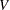. Fortables, the CHISQ option also provides Fisher’s exact test and the continuity-adjusted chi-square. See the section Chi-Square Tests and Statistics for details.

For one-way tables, the CHISQ option provides a chi-square goodness-of-fit test for equal proportions. If you specify the null hypothesis proportions with the TESTP= option, PROC FREQ computes a chi-square goodness-of-fit test for the specified proportions. If you specify null hypothesis frequencies with the TESTF= option, PROC FREQ computes a chi-square goodness-of-fit test for the specified frequencies. See the section Chi-Square Test for One-Way Tables for more information.

To request Fisher’s exact test for tables larger than, use the FISHER option in the EXACT statement. Exact tests are also available for other CHISQ statistics, including the Pearson, likelihood-ratio, and Mantel-Haenszel chi-square, and the chi-square goodness-of-fit test for one-way tables. You can use the EXACT statement to request these tests. See the section Exact Statistics for details.

You can specify the following option in parentheses following the CHISQ option:

WARN= value
WARN= ( values )

controls the warning message about the validity of the asymptotic Pearson chi-square test. By default, PROC FREQ displays a warning when more than 20% of the table cells have expected frequencies that are less than 5. If you specify the NOPRINT option in the PROC FREQ statement, this warning is included in the log; otherwise, the warning is displayed as a footnote in the chi-square table. You can use the WARN= option to suppress the warning and to include a warning indicator in the output data set.

The WARN= option can take one or more of the following values. If you specify more than one value, enclose the values in parentheses following WARN=. For example, warn = (output noprint).

Value of WARN=

Description

OUTPUT

Adds a warning indicator variable to the output data set

NOLOG

Suppresses the chi-square warning message in the log

NOPRINT

Suppresses the chi-square warning message in the display

NONE

Suppresses the chi-square warning message entirely

If you specify the WARN=OUTPUT option, the chi-square ODS output data set contains a variable named Warning that equals 1 for the Pearson chi-square when more than 20% of the table cells have expected frequencies that are less than 5 and equals 0 otherwise. If you specify WARN=OUTPUT and also specify the CHISQ option in the OUTPUT statement, then the statistics output data set contains a variable named WARN_PCHI that indicates the warning.

The WARN=NOLOG option has the same effect as the NOWARN option in the TABLES statement.

CL

requests confidence limits for the MEASURES statistics. If you omit the MEASURES option, the CL option invokes MEASURES. You can set the level of the confidence limits by using the ALPHA= option. The default of ALPHA=0.5 produces 95% confidence limits. See the sections Measures of Association and Confidence Limits for more information.

CMH < ( cmh-options ) >

requests Cochran-Mantel-Haenszel statistics, which test for association between the row and column variables after adjusting for the remaining variables in a multiway table. The Cochran-Mantel-Haenszel statistics include the nonzero correlation statistic, the row mean scores (ANOVA) statistic, and the general association statistic. In addition, fortables, the CMH option provides the adjusted Mantel-Haenszel and logit estimates of the odds ratio and relative risks, together with their confidence limits. For stratifiedtables, the CMH option provides the Breslow-Day test for homogeneity of odds ratios. (To request Tarone’s adjustment for the Breslow-Day test, specify the BDT cmh-option.) See the section Cochran-Mantel-Haenszel Statistics for details.

You can use the CMH1 or CMH2 option to control the number of CMH statistics that PROC FREQ computes.

For stratifiedtables, you can request Zelen’s exact test for equal odds ratios by specifying the EQOR option in the EXACT statement. See the section Zelen’s Exact Test for Equal Odds Ratios for details. You can request exact confidence limits for the common odds ratio by specifying the COMOR option in the EXACT statement. This option also provides a common odds ratio test. See the section Exact Confidence Limits for the Common Odds Ratio for details.

You can specify the following cmh-options in parentheses following the CMH option. These cmh-options, which apply to stratifiedtables, are also available with the CMH1 or CMH2 option.

BDT

requests Tarone’s adjustment in the Breslow-Day test for homogeneity of odds ratios. See the section Breslow-Day Test for Homogeneity of the Odds Ratios for details. The BDT cmh-option has the same effect as the BDT option in the TABLES statement.

GAILSIMON  |  GS   <COLUMN=1 | 2>

requests the Gail-Simon test for qualitative interaction, which applies to stratifiedtables. See the section Gail-Simon Test for Qualitative Interactions for details.

The COLUMN= option specifies the column of the risk differences to use in computing the Gail-Simon test. By default, PROC FREQ uses column 1 risk differences. If you specify COLUMN=2, PROC FREQ uses column 2 risk differences.

The GAILSIMON cmh-option has the same effect as the GAILSIMON option in the TABLES statement.

MANTELFLEISS  |  MF

requests the Mantel-Fleiss criterion for the Mantel-Haenszel statistic for stratifiedtables. See the section Mantel-Fleiss Criterion for details.

CMH1 < ( cmh-options ) >

requests the Cochran-Mantel-Haenszel correlation statistic. This option does not provide the CMH row mean scores (ANOVA) statistic or the general association statistic, which are provided by the CMH option. For tables larger than, the CMH1 option requires less memory than the CMH option, which can require an enormous amount of memory for large tables.

Fortables, the CMH1 option also provides the adjusted Mantel-Haenszel and logit estimates of the odds ratio and relative risks, together with their confidence limits. For stratifiedtables, the CMH1 option provides the Breslow-Day test for homogeneity of odds ratios.

The cmh-options available with the CMH1 option are the same as those available with the CMH option. See the description of the CMH option for details.

CMH2 < ( cmh-options ) >

requests the Cochran-Mantel-Haenszel correlation statistic and the row mean scores (ANOVA) statistic. This option does not provide the CMH general association statistic, which is provided by the CMH option. For tables larger than, the CMH2 option requires less memory than the CMH option, which can require an enormous amount of memory for large tables.

Fortables, the CMH1 option also provides the adjusted Mantel-Haenszel and logit estimates of the odds ratio and relative risks, together with their confidence limits. For stratifiedtables, the CMH1 option provides the Breslow-Day test for homogeneity of odds ratios.

The cmh-options available with the CMH2 option are the same as those available with the CMH option. See the description of the CMH option for details.

CONTENTS='string'

specifies the label to use for crosstabulation tables in the contents file, the Results window, and the trace record. For information about output presentation, see the SAS Output Delivery System: User's Guide.

If you omit the CONTENTS= option, the contents label for crosstabulation tables is "Cross-Tabular Freq Table" by default.

Note that contents labels for all crosstabulation tables that are produced by a single TABLES statement use the same text. To specify different contents labels for different crosstabulation tables, request the tables in separate TABLES statements and use the CONTENTS= option in each TABLES statement.

To remove the crosstabulation table entry from the contents file, you can specify a null label with CONTENTS=''.

The CONTENTS= option affects only contents labels for crosstabulation tables. It does not affect contents labels for other PROC FREQ tables.

To specify the contents label for any PROC FREQ table, you can use PROC TEMPLATE to create a customized table definition. The CONTENTS_LABEL attribute in the DEFINE TABLE statement of PROC TEMPLATE specifies the contents label for the table. See the chapter "The TEMPLATE Procedure" in the SAS Output Delivery System: User's Guide for more information.

CONVERGE=value

specifies the convergence criterion for computing the polychoric correlation, which you request with the PLCORR option. The CONVERGE= value must be a positive number. By default CONVERGE=0.0001. Iterative computation of the polychoric correlation stops when the convergence measure falls below the value of CONVERGE= or when the number of iterations exceeds the value specified in the MAXITER= option, whichever happens first. See the section Polychoric Correlation for details.

CROSSLIST

displays crosstabulation tables in ODS column format instead of the default crosstabulation cell format. In a CROSSLIST table display, the rows correspond to the crosstabulation table cells, and the columns correspond to descriptive statistics such as Frequency and Percent. The CROSSLIST table displays the same information as the default crosstabulation table, but uses an ODS column format instead of the table cell format. See the section Multiway Tables for details about the contents of the CROSSLIST table.

You can control the contents of a CROSSLIST table with the same options available for the default crosstabulation table. These include the NOFREQ, NOPERCENT, NOROW, and NOCOL options. You can request additional information in a CROSSLIST table with the CELLCHI2, DEVIATION, EXPECTED, MISSPRINT, and TOTPCT options.

The FORMAT= option and the CUMCOL option have no effect for CROSSLIST tables. You cannot specify both the LIST option and the CROSSLIST option in the same TABLES statement.

You can use the NOSPARSE option to suppress display of variable levels with zero frequency in CROSSLIST tables. By default for CROSSLIST tables, PROC FREQ displays all levels of the column variable within each level of the row variable, including any column variable levels with zero frequency for that row. And for multiway tables displayed with the CROSSLIST option, the procedure displays all levels of the row variable for each stratum of the table by default, including any row variable levels with zero frequency for the stratum.

CUMCOL

displays the cumulative column percentages in the cells of the crosstabulation table. The CUMCOL option does not apply to crosstabulation tables produced with the LIST or CROSSLIST option.

DEVIATION

displays the deviation of the frequency from the expected frequency for each cell of the crosstabulation table. See the section Pearson Chi-Square Test for Two-Way Tables for details. The DEVIATION option does not apply to crosstabulation tables produced with the LIST option.

EXPECTED

displays the expected cell frequencies under the hypothesis of independence (or homogeneity) for crosstabulation tables. See the section Pearson Chi-Square Test for Two-Way Tables for details. The EXPECTED option does not apply to tables produced with the LIST option.

FISHER | EXACT

requests Fisher’s exact test for tables that are larger than. (Fortables, the CHISQ option provides Fisher’s exact test.) This test is also known as the Freeman-Halton test. See the sections Fisher’s Exact Test and Exact Statistics for more information.

If you omit the CHISQ option in the TABLES statement, the FISHER option invokes CHISQ. You can also request Fisher’s exact test by specifying the FISHER option in the EXACT statement.

Note:PROC FREQ computes exact tests with fast and efficient algorithms that are superior to direct enumeration. Exact tests are appropriate when a data set is small, sparse, skewed, or heavily tied. For some large problems, computation of exact tests might require a considerable amount of time and memory. Consider using asymptotic tests for such problems. Alternatively, when asymptotic methods might not be sufficient for such large problems, consider using Monte Carlo estimation of exact p-values. See the section Computational Resources for more information.

FORMAT=format-name

specifies a format for the following crosstabulation table cell values: frequency, expected frequency, and deviation. PROC FREQ also uses the specified format to display the row and column total frequencies and the overall total frequency in crosstabulation tables.

You can specify any standard SAS numeric format or a numeric format defined with the FORMAT procedure. The format length must not exceed 24. If you omit the FORMAT= option, by default PROC FREQ uses the BEST6. format to display frequencies less than 1E6, and the BEST7. format otherwise.

The FORMAT= option applies only to crosstabulation tables displayed in the default format. It does not apply to crosstabulation tables produced with the LIST or CROSSLIST option.

To change display formats in any FREQ table, you can use PROC TEMPLATE. See the chapter "The TEMPLATE Procedure" in the SAS Output Delivery System: User's Guide for more information.

GAILSIMON   |   GS   <COLUMN = 1 | 2>

requests the Gail-Simon test for qualitative interaction, which applies to stratifiedtables. See the section Gail-Simon Test for Qualitative Interactions for details.

The COLUMN= option specifies the column of the risk differences to use in computing the Gail-Simon test. By default, PROC FREQ uses column 1 risk differences. If you specify COLUMN=2, PROC FREQ uses column 2 risk differences.

JT

requests the Jonckheere-Terpstra test. See the section Jonckheere-Terpstra Test for details.

LIST

displays two-way to-way crosstabulation tables in a list format instead of the default crosstabulation cell format. The LIST option displays the entire multiway table in one table, instead of displaying a separate two-way table for each stratum. See the section Multiway Tables for details.

The LIST option is not available when you also specify statistical options. You must use the standard crosstabulation table display or the CROSSLIST display when you request statistical tests or measures.

MAXITER=number

specifies the maximum number of iterations for computing the polychoric correlation, which you request with the PLCORR option. The value of the MAXITER= option must be a positive integer. By default MAXITER=20. Iterative computation of the polychoric correlation stops when the number of iterations exceeds the MAXITER= value or when the convergence measures falls below the value of the CONVERGE= option, whichever happens first. See the section Polychoric Correlation for details.

MEASURES

requests several measures of association and their asymptotic standard errors. The MEASURES option provides the following statistics: gamma, Kendall’s tau-, Stuart’s tau-, Somers’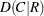, Somers’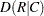, the Pearson and Spearman correlation coefficients, lambda (symmetric and asymmetric), and uncertainty coefficients (symmetric and asymmetric). To request confidence limits for these measures of association, you can specify the CL option.

Fortables, the MEASURES option also provides the odds ratio, column 1 relative risk, column 2 relative risk, and the corresponding confidence limits. Alternatively, you can obtain the odds ratio and relative risks, without the other measures of association, by specifying the RELRISK option.

See the section Measures of Association for details.

You can use the TEST statement to request asymptotic tests for the following measures of association: gamma, Kendall’s tau-, Stuart’s tau-, Somers’, Somers’, and the Pearson and Spearman correlation coefficients. You can use the EXACT statement to request exact confidence limits for the odds ratio and exact tests for the following measures of association: Kendall’s tau-, Stuart’s tau-, Somers’and, and the Pearson and Spearman correlation coefficients. See the section Exact Statistics for more information.

MISSING

treats missing values as a valid nonmissing level for all TABLES variables. The MISSING option displays the missing levels in frequency and crosstabulation tables and includes them in all calculations of percentages, tests, and measures.

By default, if you do not specify the MISSING or MISSPRINT option, an observation is excluded from a table if it has a missing value for any of the variables in the TABLES request. When PROC FREQ excludes observations with missing values, it displays the total frequency of missing observations below the table. See the section Missing Values for more information.

MISSPRINT

displays missing value frequencies in frequency and crosstabulation tables but does not include the missing value frequencies in any computations of percentages, tests, or measures.

By default, if you do not specify the MISSING or MISSPRINT option, an observation is excluded from a table if it has a missing value for any of the variables in the TABLES request. When PROC FREQ excludes observations with missing values, it displays the total frequency of missing observations below the table. See the section Missing Values for more information.

NOCOL

suppresses the display of column percentages in crosstabulation table cells.

NOCUM

suppresses the display of cumulative frequencies and percentages in one-way frequency tables. The NOCUM option also suppresses the display of cumulative frequencies and percentages in crosstabulation tables in list format, which you request with the LIST option.

NOFREQ

suppresses the display of cell frequencies in crosstabulation tables. The NOFREQ option also suppresses row total frequencies. This option has no effect for one-way tables or for crosstabulation tables in list format, which you request with the LIST option.

NOPERCENT

suppresses the display of overall percentages in crosstabulation tables. These percentages include the cell percentages of the total (two-way) table frequency, as well as the row and column percentages of the total table frequency. To suppress the display of cell percentages of row or column totals, use the NOROW or NOCOL option, respectively.

For one-way frequency tables and crosstabulation tables in list format, the NOPERCENT option suppresses the display of percentages and cumulative percentages.

NOPRINT

suppresses the display of frequency and crosstabulation tables but displays all requested tests and statistics. To suppress the display of all output, including tests and statistics, use the NOPRINT option in the PROC FREQ statement.

NOROW

suppresses the display of row percentages in crosstabulation table cells.

NOSPARSE

suppresses the display of cells with a zero frequency count in LIST output and omits them from the OUT= data set. The NOSPARSE option applies when you specify the ZEROS option in the WEIGHT statement to include observations with zero weights. By default, the ZEROS option invokes the SPARSE option, which displays table cells with a zero frequency count in the LIST output and includes them in the OUT= data set. See the description of the ZEROS option for more information.

The NOSPARSE option also suppresses the display of variable levels with zero frequency in CROSSLIST tables. By default for CROSSLIST tables, PROC FREQ displays all levels of the column variable within each level of the row variable, including any column variable levels with zero frequency for that row. For multiway tables displayed with the CROSSLIST option, the procedure displays all levels of the row variable for each stratum of the table by default, including any row variable levels with zero frequency for the stratum.

NOWARN

suppresses the log warning message about the validity of the asymptotic Pearson chi-square test. By default, PROC FREQ provides a warning about the validity of the asymptotic Pearson chi-square test when more than 20cells have expected frequencies that are less than 5. This warning message appears in the log if you specify the NOPRINT option in the PROC FREQ statement,

The NOWARN option is equivalent to the CHISQ(WARN=NOLOG) option. You can also use the CHISQ(WARN=) option to suppress the warning message in the display and to request a warning variable in the chi-square ODS output data set or in the OUTPUT data set.

OUT=SAS-data-set

names an output data set that contains frequency or crosstabulation table counts and percentages. If more than one table request appears in the TABLES statement, the contents of the OUT= data set correspond to the last table request in the TABLES statement. The OUT= data set variable COUNT contains the frequencies and the variable PERCENT contains the percentages. See the section Output Data Sets for details. You can specify the following options to include additional information in the OUT= data set: OUTCUM, OUTEXPECT, and OUTPCT.

OUTCUM

includes cumulative frequencies and cumulative percentages in the OUT= data set for one-way tables. The variable CUM_FREQ contains the cumulative frequencies, and the variable CUM_PCT contains the cumulative percentages. See the section Output Data Sets for details. The OUTCUM option has no effect for two-way or multiway tables.

OUTEXPECT

includes expected cell frequencies in the OUT= data set for crosstabulation tables. The variable EXPECTED contains the expected cell frequencies. See the section Output Data Sets for details. The EXPECTED option has no effect for one-way tables.

OUTPCT

includes the following additional variables in the OUT= data set for crosstabulation tables:

PCT_COL

percentage of column frequency

PCT_ROW

percentage of row frequency

PCT_TABL

percentage of stratum (two-way table) frequency, for-way tables whereSee the section Output Data Sets for details. The OUTPCT option has no effect for one-way tables.

PLCORR

requests the polychoric correlation coefficient. Fortables, this statistic is more commonly known as the tetrachoric correlation coefficient, and it is labeled as such in the displayed output. See the section Polychoric Correlation for details. Also see the descriptions of the CONVERGE= and MAXITER= options, which you can specify to control the iterative computation of the polychoric correlation coefficient.

If you omit the MEASURES option, the PLCORR option invokes MEASURES.

PLOTS < ( global-plot-options ) > < = plot-request < ( plot-options ) > >
PLOTS < ( global-plot-options ) >
< = ( plot-request < (plot-options) > <...plot-request < (plot-options ) >> )>

controls the plots that are produced through ODS Graphics. Plot-requests identify the plots, and plot-options control the appearance and content of the plots. You can specify plot-options in parentheses following a plot-request. A global-plot-option applies to all plots for which it is available, unless it is altered by a specific plot-option. You can specify global-plot-options in parentheses following the PLOTS option.

When you specify only one plot-request, you can omit the parentheses around the request. For example:

``` plots=all
plots=freqplot
plots=(freqplot oddsratioplot)
plots(only)=(cumfreqplot deviationplot)
```

For information about ODS Graphics, see Chapter 21, Statistical Graphics Using ODS (SAS/STAT 9.22 User's Guide). You must enable ODS Graphics before requesting plots, as shown in the following example:

```ods graphics on;
proc freq;
tables treatment*response / chisq plots=freqplot;
weight wt;
run;
ods graphics off;
```

The PLOTS= option has no effect when you specify the NOPRINT option in the PROC FREQ statement.

If you have enabled ODS Graphics but do not specify the PLOTS= option, then PROC FREQ produces all plots that are associated with the analyses that you request in the TABLES statement.

Table 3.11 lists the available plot-requests, together with their plot-options and required TABLES statement options.

Table 3.11 PLOTS= Options

Plot-Request

Plot-Options

Required TABLES Statement Option

One-way table request

CHISQ (one-way table)

Any table request

Two-way or multiway table

AGREE (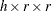table)

EXACT(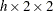table)

(table)

EXACT(table)

(table,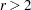)

Global-Plot-Options

A global-plot-option applies to all plots for which the option is available, unless it is altered by a specific plot-option. You can specify global-plot-options in parentheses following the PLOTS option.

The following specific plot-options are available as global-plot-options: COLUMN=, EXACT, LOGBASE=, NPANELPOS=, ORDER=, ORIENT= RANGE=, SCALE=, STATS, and TYPE=. These plot-options are described in the section "Plot-Options." Additionally, you can specify the following global-plot-option in parentheses following the PLOTS option:

ONLY

suppresses the default plots and requests only the plots that are specified as plot-requests.

Plot-Requests

The following plot-requests are available with the PLOTS= option:

ALL

requests all plots that are associated with the specified analyses. This is the default if you do not specify the PLOTS(ONLY) option.

CUMFREQPLOT < ( plot-options ) >

requests a plot of cumulative frequencies. Cumulative frequency plots are available for one-way frequency tables. The following plot-options are available for CUMFREQPLOT: ORIENT=, SCALE=, and TYPE=.

DEVIATIONPLOT < ( plot-options ) >

requests a plot of relative deviations from expected frequencies. Deviation plots are available for one-way frequency tables. To produce a deviation plot, you must also specify the CHISQ option in the TABLES statement. The following plot-options are available for DEVIATIONPLOT: NOSTAT, ORIENT= and TYPE=.

FREQPLOT < ( plot-options ) >

requests a frequency plot. Frequency plots are available for frequency and crosstabulation tables. For multiway tables, PROC FREQ provides a two-way frequency plot for each stratum.

The following plot-options are available for FREQPLOT for all tables: ORIENT=, SCALE=, and TYPE=. Additionally, the TWOWAY= and NPANELPOS= plot-options are available for two-way and multiway tables. You can use the TWOWAY= plot-option to specify the layout of a two-way frequency plot. The NPANELPOS= plot-option is not available with the TWOWAY=STACKED layout.

KAPPAPLOT < ( plot-options ) >

requests a plot of kappa statistics and confidence limits. Kappa plots are available for multiway square tables. To produce a kappa plot, you must also specify the AGREE option in the TABLES statement. The following plot-options are available for KAPPAPLOT: NPANELPOS=, ORDER=, RANGE=, and STATS.

NONE

suppresses all plots.

ODDSRATIOPLOT < ( plot-options ) >

requests a plot of odds ratios with confidence limits. Odds ratio plots are available for multiwaytables. To produce an odds ratio plot, you must also specify the MEASURES or RELRISK option in the TABLES statement. The following plot-options are available for ODDSRATIOPLOT: EXACT, LOGBASE=, NPANELPOS=, ORDER=, RANGE=, and STATS. If you request a plot of exact confidence limits by specifying the EXACT plot-option, you must also request computation of exact confidence limits by specifying the EXACT statement.

RELRISKPLOT < ( plot-options ) >

requests a plot of relative risks with confidence limits. Relative risk plots are available for multiwaytables. To produce a relative risk plot, you must also specify the MEASURES or RELRISK option in the TABLES statement. The following plot-options are available for RELRISKPLOT: COLUMN=, LOGBASE=, NPANELPOS=, ORDER=, RANGE=, and STATS.

RISKDIFFPLOT < ( plot-options ) >

requests a plot of risk (proportion) differences with confidence limits. Risk difference plots are available for multiwaytables. To produce a risk difference plot, you must also specify the RISKDIFF option in the TABLES statement. The following plot-options are available for RISKDIFF: COLUMN=, EXACT, NPANELPOS=, ORDER=, RANGE=, and STATS. If you request a plot of exact confidence limits by specifying the EXACT plot-option, you must also request computation of exact confidence limits by specifying the EXACT statement.

WTKAPPAPLOT < ( plot-options ) >

requests a plot of weighted kappa statistics with confidence limits. Weighted kappa plots are available for multiway square tables. To produce a weighted kappa plot, you must also specify the AGREE option in the TABLES statement. Note that simple kappa and weighted kappa statistics are the same fortables; therefore, the procedure does not present weighted kappa statistics fortables. The following plot-options are available for WTKAPPAPLOT: NPANELPOS=, ORDER=, RANGE=, and STATS.

Plot-Options

You can specify the following plot-options in parentheses after a plot-request:

COLUMN=1 | 2

specifies the risk column for the relative risk plot (RELRISKPLOT) and the risk difference plot (RISKDIFFPLOT). If you specify the COLUMN=1 plot-option, then the plot displays the column 1 risk differences or the column 1 relative risks. Similarly, COLUMN=2 displays the column 2 risk differences or relative risks. The default is COLUMN=1.

EXACT

requests exact confidence limits instead of asymptotic confidence limits in the odds ratio plot (ODDSRATIOPLOT) and the risk difference plot (RISKDIFFPLOT). If you specify the EXACT plot-option, you must also request computation of the exact confidence limits by specifying the EXACT statement.

LOGBASE=2 | E | 10

applies to the odds ratio plot (ODDSRATIOPLOT) and the relative risk plot (RELRISKPLOT). The LOGBASE= plot-option displays the odds ratio or relative risk axis on the specified log scale.

NOSTAT

applies to the deviation plot (DEVIATIONPLOT). The NOSTAT plot-option suppresses the chi-square p-value that is displayed by default in the deviation plot.

NPANELPOS=n

applies to the following plots: FREQPLOT (for two-way and multiway tables), KAPPAPLOT, ODDSRATIOPLOT, RELRISKPLOT, RISKDIFFPLOT, and WTKAPPAPLOT.

The NPANELPOS= plot-option divides the plot into multiple panels that display at most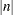statistics per panel. Ifis positive, the number of statistics per panel is balanced; but ifis negative, the number of statistics per panel is not balanced. By default,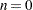and all statistics are displayed in a single plot. For example, suppose you want to display 21 odds ratios. Then NPANELPOS=20 displays two panels, the first with 11 odds ratios and the second with 10; NPANELPOS=–20 displays 20 odds ratios in the first panel but only one in the second.

For two-way frequency plots, NPANELPOS= divides the plot into multiple panels that display at mostlevels of the row variable per panel. The NPANELPOS= plot-option applies to two-way plots that are displayed with grouped layout, which you specify with the TWOWAY=GROUPVERTICAL or TWOWAY=GROUPHORIZONTAL plot-option. The NPANELPOS= plot-option does not apply to the TWOWAY=STACKED layout.

ORDER=ASCENDING | DESCENDING

displays the statistics in sorted order. By default, the statistics are displayed in the order in which the corresponding strata appear in the multiway table display. The ORDER= plot-option applies to the following plots: KAPPAPLOT, ODDSRATIOPLOT, RELRISKPLOT, RISKDIFFPLOT, and WTKAPPAPLOT.

ORIENT=HORIZONTAL | VERTICAL

controls the orientation of the plot. The ORIENT= plot-option applies to the following plots: CUMFREQPLOT, DEVIATIONPLOT, and FREQPLOT.

ORIENT=HORIZONTAL places the variable levels on the Y axis and the frequencies, percentages, or statistic-values on the X axis. ORIENT=VERTICAL places the variable levels on the X axis. The default orientation is ORIENT=VERTICAL for bar charts (TYPE=BAR) and ORIENT=HORIZONTAL for dot plots (TYPE=DOT).

RANGE= ( <min> <,max> )  | CLIP

specifies the range of values to display. The RANGE= plot-option applies to the following plots: KAPPAPLOT, ODDSRATIOPLOT, RELRISKPLOT, RISKDIFFPLOT, and WTKAPPAPLOT. If you specify RANGE=CLIP, the confidence limits are clipped and the display range is determined by the minimum and maximum values of the estimates. By default, the display range includes all confidence limits.

SCALE=FREQ | LOG | PERCENT| SQRT

specifies the scale of the frequencies to display. The SCALE= plot-option applies to the frequency plot (FREQPLOT) and the cumulative frequency plot (CUMFREQPLOT).

The default is SCALE=FREQ, which displays unscaled frequencies. SCALE=LOG displays log (base 10) frequencies. SCALE=PERCENT displays percentages (relative frequencies). SCALE=SQRT displays square roots of the frequencies, which produces a plot known as a rootogram.

STATS

displays the values of the statistics and their confidence limits on the right side of the plot. If you do not request the STATS plot-option, the statistic values are not displayed. The STATS plot-option applies to the following plots: KAPPAPLOT, ODDSRATIOPLOT, RELRISKPLOT, RISKDIFFPLOT, and WTKAPPAPLOT.

TWOWAY=GROUPVERTICAL | GROUPHORIZONTAL | STACKED

specifies the layout for a two-way frequency plot (FREQPLOT). The TWOWAY= plot-option applies to frequency plots for two-way and multiway table requests; PROC FREQ produces a two-way frequency plot for each stratum of a multiway table request.

TWOWAY=GROUPVERTICAL produces a grouped plot with a vertical common baseline. The plot is grouped by the row variable, which is the first variable that you specify in a two-way table request. TWOWAY=GROUPHORIZONTAL produces a grouped plot with a horizontal common baseline.

TWOWAY=STACKED produces stacked frequency plots for two-way tables. In a stacked bar chart, the bars correspond to the column variable values, and the row frequencies are stacked within each column. In a stacked dot plot, the dotted lines correspond to the columns, and the row frequencies within columns are plotted as data dots on the same column line.

The default two-way layout is TWOWAY=GROUPVERTICAL. The ORIENT=, SCALE=, and TYPE= plot-options are available for each TWOWAY= layout.

TYPE=BARCHART | DOTPLOT

specifies the plot type for frequency (FREQPLOT), cumulative frequency (CUMFREQPLOT), and deviation plots (DEVIATIONPLOT). TYPE=BARCHART produces a bar chart, and TYPE=DOTPLOT produces a dot plot. The default is TYPE=BARCHART.

PRINTKWT

displays the weights that PROC FREQ uses to compute the weighted kappa coefficient. You must also specify the AGREE option to request the weighted kappa coefficient. You can specify (WT=FC) with the AGREE option to request Fleiss-Cohen weights. By default, PROC FREQ uses Cicchetti-Allison weights to compute the weighted kappa coefficient. See the section Weighted Kappa Coefficient for details.

RELRISK

requests relative risk measures and their confidence limits fortables. These measures include the odds ratio and the column 1 and 2 relative risks. See the section Odds Ratio and Relative Risks for 2 x 2 Tables for details.

You can also obtain the RELRISK measures by specifying the MEASURES option, which produces other measures of association in addition to the relative risks.

You can request exact confidence limits for the odds ratio by specifying the OR option in the EXACT statement.

RISKDIFF <(riskdiff-options)>

requests risks, or binomial proportions, fortables. For column 1 and column 2, PROC FREQ computes the row 1 risk, row 2 risk, total risk, and risk difference (row 1 – row 2), together with their asymptotic standard errors and asymptotic (Wald) confidence limits. PROC FREQ also provides exact (Clopper-Pearson) confidence limits for the row 1, row 2, and total risks. The ALPHA= option determines the confidence level, and the default of ALPHA=0.05 produces 95% confidence limits. See the section Risks and Risk Differences for details.

You can specify riskdiff-options inside the parentheses following the RISKDIFF option to request tests of noninferiority, superiority, and equivalence for the risk difference. Available test methods include Farrington-Manning, Hauck-Anderson, and Newcombe score (Wilson), in addition to the Wald test. Table 3.12 summarizes the riskdiff-options.

You can request exact unconditional confidence limits for the risk difference by specifying the RISKDIFF option in the EXACT statement. See the section Exact Unconditional Confidence Limits for the Risk Difference for more information.

Table 3.12 RISKDIFF (Proportion Difference) Options

Task

Riskdiff-Option

Specify the column

Request a continuity correction

Request tests

Specify the test method

Specify the test margin

Specify the test variance

You can specify the following riskdiff-options inside parentheses following the RISKDIFF option.

COLUMN=1 | 2 | BOTH

specifies the table column for which to compute the risk difference tests of noninferiority, superiority, or equivalence, which you request with the NONINF, SUP, and EQUIV riskdiff-options, respectively. You can specify COLUMN=1, COLUMN=2, or COLUMN=BOTH. If you do not specify the COLUMN= option, PROC FREQ computes the risk difference tests for column 1. The COLUMN= option has no effect on the table of risk estimates and confidence limits or on the equality test; PROC FREQ computes these statistics for both column 1 and column 2.

CORRECT

includes a continuity correction in the asymptotic Wald confidence limits and tests. The CORRECT riskdiff-option also includes a continuity correction in the Newcombe score confidence limits, which you request with the METHOD=SCORE riskdiff-option. METHOD=HA and METHOD=FM do not use continuity corrections. The CORRECT riskdiff-option has the same effect as the RISKDIFFC option.

EQUAL

requests a test of the null hypothesis that the risk difference equals zero. PROC FREQ provides an asymptotic Wald test of equality. See the section Equality Test for details. You can specify the test variance type with the VAR= riskdiff-option.

EQUIV | EQUIVALENCE

requests a test of equivalence for the risk difference. See the section Equivalence Tests for details. You can specify the equivalence test margins with the MARGIN= riskdiff-option and the test method with the METHOD= riskdiff-option. PROC FREQ uses METHOD=WALD by default.

MARGIN=value | (lower,upper)

specifies the margin for the noninferiority, superiority, and equivalence tests, which you request with the NONINF, SUP, and EQUIV riskdiff-options, respectively. If you do not specify MARGIN=, PROC FREQ uses a margin of 0.2 by default.

For noninferiority and superiority tests, specify a single value for the MARGIN= riskdiff-option. The MARGIN= value must be a positive number. You can specify value as a number between 0 and 1. Or you can specify value in percentage form as a number between 1 and 100, and PROC FREQ converts that number to a proportion. The procedure treats the value 1 as 1%.

For an equivalence test, you can specify a single MARGIN= value, or you can specify both lower and upper values. If you specify a single MARGIN= value, it must be a positive number, as described previously. If you specify a single MARGIN= value for an equivalence test, PROC FREQ uses –value as the lower margin and value as the upper margin for the test. If you specify both lower and upper values for an equivalence test, you can specify them in proportion form as numbers between –1 or 1. Or you can specify them in percentage form as numbers between –100 and 100, and PROC FREQ converts the numbers to proportions. The value of lower must be less than the value of upper.

METHOD=method

specifies the method for the noninferiority, superiority, and equivalence tests, which you request with the NONINF, SUP, and EQUIV riskdiff-options, respectively. The following methods are available:

FM

Farrington-Manning

HA

Hauck-Anderson

SCORE | NEWCOMBE | WILSON

Newcombe score (Wilson)

WALD

Wald

The default is METHOD=WALD. See the section Noninferiority Test for descriptions of these methods.

For METHOD=SCORE and METHOD=WALD, you can request a continuity correction with the CORRECT riskdiff-option. For METHOD=WALD, you can specify the variance type with the VAR= riskdiff-option.

NONINF | NONINFERIORITY

requests a test of noninferiority for the risk difference. See the section Noninferiority Test for details. You can specify the test margin with the MARGIN= riskdiff-option and the test method with the METHOD= riskdiff-option. PROC FREQ uses METHOD=WALD by default.

SUP | SUPERIORITY

requests a test of superiority for the binomial proportion. See the section Superiority Test for details. You can specify the test margin with the MARGIN= riskdiff-option and the test method with the METHOD= riskdiff-option. PROC FREQ uses METHOD=WALD by default.

VAR=SAMPLE | NULL

specifies the type of variance estimate to use for the Wald tests of noninferiority, superiority, equivalence, and equality. The default is VAR=SAMPLE, which estimates the variance from the sample proportions. VAR=NULL uses the null hypothesis values to compute the variance. See the sections Equality Test and Noninferiority Test for details.

RISKDIFFC <(riskdiff-options)>

requests the RISKDIFF option statistics fortables and includes a continuity correction in the asymptotic Wald confidence limits and tests.

The RISKDIFF option statistics include risks, or binomial proportions, fortables. For column 1 and column 2, PROC FREQ computes the row 1 risk, row 2 risk, total risk, and risk difference (row 1 – row 2), together with their asymptotic standard errors and asymptotic (Wald) confidence limits. PROC FREQ also provides exact (Clopper-Pearson) confidence limits for the row 1, row 2, and total risks. See the section Risks and Risk Differences for details.

You can request additional tests and statistics for the risk difference by specifying riskdiff-options in parentheses after RISKDIFFC. The riskdiff-options are the same as those available with RISKDIFF. See the description of the RISKDIFF option for details.

You can request exact unconditional confidence limits for the risk difference by specifying the RISKDIFF option in the EXACT statement. See the section Exact Unconditional Confidence Limits for the Risk Difference for more information.

SCORES=type

specifies the type of row and column scores that PROC FREQ uses to compute the following statistics: Mantel-Haenszel chi-square, Pearson correlation, Cochran-Armitage test for trend, weighted kappa coefficient, and Cochran-Mantel-Haenszel statistics. The value of type can be one of the following:

• MODRIDIT

• RANK

• RIDIT

• TABLE

See the section Scores for descriptions of these score types.

If you do not specify the SCORES= option, PROC FREQ uses SCORES=TABLE by default. For character variables, the row and column TABLE scores are the row and column numbers. That is, the TABLE score is 1 for row 1, 2 for row 2, and so on. For numeric variables, the row and column TABLE scores equal the variable values. See the section Scores for details. Using MODRIDIT, RANK, or RIDIT scores yields nonparametric analyses.

You can use the SCOROUT option to display the row and column scores.

SCOROUT

displays the row and column scores that PROC FREQ uses to compute score-based tests and statistics. You can specify the score type with the SCORES= option. See the section Scores for details.

The scores are computed and displayed only when PROC FREQ computes statistics for two-way tables. You can use ODS to store the scores in an output data set. See the section ODS Table Names for more information.

SPARSE

reports all possible combinations of the variable values for an n-way table when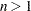, even if a combination does not occur in the data. The SPARSE option applies only to crosstabulation tables displayed in LIST format and to the OUT= output data set. If you do not use the LIST or OUT= option, the SPARSE option has no effect.

When you specify the SPARSE and LIST options, PROC FREQ displays all combinations of variable values in the table listing, including those with a frequency count of zero. By default, without the SPARSE option, PROC FREQ does not display zero-frequency levels in LIST output. When you use the SPARSE and OUT= options, PROC FREQ includes empty crosstabulation table cells in the output data set. By default, PROC FREQ does not include zero-frequency table cells in the output data set.

See the section Missing Values for more information.

TESTF=(values)

specifies the null hypothesis frequencies for a one-way chi-square goodness-of-fit test, which you request with the CHISQ option. See the section Chi-Square Test for One-Way Tables for details.

You can separate the TESTF= values with blanks or commas. The number of values must equal the number of variable levels in the one-way table. The sum of the values must equal the total frequency for the one-way table. List the values in the order in which the corresponding variable levels appear in the output. If you omit the CHISQ option, the TESTF= option invokes CHISQ.

TESTP=(values)

specifies the null hypothesis proportions for a one-way chi-square goodness-of-fit test, which you request with the CHISQ option. See the section Chi-Square Test for One-Way Tables for details.

You can separate the TESTP= values with blanks or commas. The number of values must equal the number of variable levels in the one-way table. List the values in the order in which the corresponding variable levels appear in the output. You can specify values in probability form as numbers between 0 and 1, where the proportions sum to 1. Or you can specify values in percentage form as numbers between 0 and 100, where the percentages sum to 100. If you omit the CHISQ option, the TESTP= option invokes CHISQ.

TOTPCT

displays the percentage of the total multiway table frequency in crosstabulation tables for-way tables, where. By default, PROC FREQ displays the percentage of the individual two-way table frequency but does not display the percentage of the total frequency for multiway crosstabulation tables. See the section Multiway Tables for more information.

The percentage of total multiway table frequency is displayed by default when you specify the LIST option. It is also provided by default in the PERCENT variable in the OUT= output data set.

TREND

requests the Cochran-Armitage test for trend. The table must be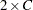or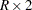to compute the trend test. See the section Cochran-Armitage Test for Trend for details.Previous Page | Next Page | Top of Page